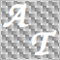• Información general
• Comentarios (1)
• Discusión

# Variable Index Dynamic Average

The Variable Index Dynamic Average (VIDyA) technical indicator has been developed by Tushar Chande. It is an original calculation method of the exponential moving average (EMA) with dynamically changing averaging period. Period of averaging depends on the market volatility; as the measure of volatility Chande Momentum Oscillator (CMO) was chosen. This oscillator measures the ratio between the sum of positive increments and sum of negative increments for a certain period (CMO period). CMO value is used as the ratio to the smoothing factor EMA. The VIDYA indicator has two main configuration parameters: the CMO oscillator parameter and the smoothing parameter of the exponential moving average (EMA period).

### Parameters

• Period CMO - the period of the oscillator
• Period EMA - the period of the moving average
• Indicator's shift - horizontal shift of the indicator in bars